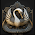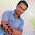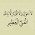# Quantitative Aptitude Questions and Answers PDF

In the test of Quantitative Aptitude, 50 questions are asked. Every question is objective type with 5 multiple answer ch0ices. Even though the syllabus is not well-defined in these competitive examinations, yet the questions asked in various earlier exams themselves define the pattern, standard etc. of the test. Observation of questions reveals that about 5O% of them are based on Data Interpretation and Data Sufficiencey. Remaining 5O% of questions are based on chapters of
1. Simplification, 2. HCF & LCM, 3. Average, 4. Percentage, 5. Ratio & Proportion, 6. Pr0fit & Loss, 7. Time & Distance, 8. Time & Work, 9. Compound Interest, 10. Simple Interest, 11. Area of Plane Figures, 12. Number Series, 13. Volume, 14. Approximation, 15. Permutation & Combination, 16. Probability and Quadratic 17. Equations & Inequations, 18. Alligation on Mixture, 19. Area & Perimeter, 20. Volume and Surface Areas.

Generally, The questions are concept based. It needs little effort to be Solved. Some t0pics appear unfamiliar to students with non-mathematical background. For example, topics like Permutation and Combination, Probability are certainly out of the scope of Arithmetic, This is the outcome of hard competition and demand of time. Other topics like Data Interpretation, Data Sufficiency are nothing but an enlargement of scope of Arithmetic and use of Arithmetical tools like Percentage, Average, Rati0 etc.

Quantitative Aptitude - Arithmetic - Numerical Ability (Questions and Answers Type)
Simple Arithmetic Computations - Download
Average - Download
Ratio and Proportion - Download
Time and Distance - Download
Time and Work - Download
Partnership - Download
Percentage - Download
Finding Wrong Numbers - Download
Quantitative Aptitude Questions & Answers (with explanations) - Model Practice Test 1 | Test 2 | Test 3

Consider following facts in course of exam preparation:
• Analyse the questions of previous exams quantitatively and qualitatively. This will give a clear view of the questions of the said examination
• Never read Mathematics. It means start Mathematics with pen and paper.
• Emphasize on concept building
• Believe in continuous rigorous practice. It will consolidate your confidence and help in Time Management by improving speed and accuracy, the two gems of success in today's exams.
• Self-evaluate yourself regularly
Posted by - Blog Author: 1:03 AM Last Updated at: 1:03 AM

#### 11 comments:

1.THANK UUUUUUUUUUUUUUUUUUUUUUUUUUUUUUUUUUUUUUU SO much

2.Thank uuuu So Much

3.thank uuuuuuuuuuuuu sir

4.thank uuuuuuuuuuuu

5.very nice web for students

6.7.8.9.in tnpsc exam ,25 qns only asked from aptitude

10.So helpful thanks

11.Thanks for your guidance and very useful for your question patterns